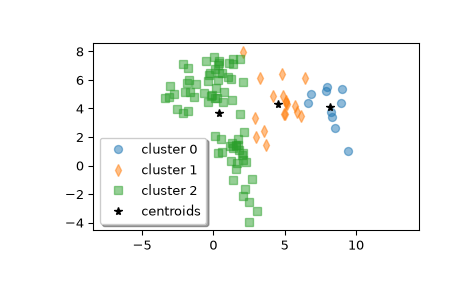# scipy.cluster.vq.kmeans2¶

scipy.cluster.vq.kmeans2(data, k, iter=10, thresh=1e-05, minit='random', missing='warn', check_finite=True)[source]

Classify a set of observations into k clusters using the k-means algorithm.

The algorithm attempts to minimize the Euclidean distance between observations and centroids. Several initialization methods are included.

Parameters
datandarray

A ‘M’ by ‘N’ array of ‘M’ observations in ‘N’ dimensions or a length ‘M’ array of ‘M’ 1-D observations.

kint or ndarray

The number of clusters to form as well as the number of centroids to generate. If minit initialization string is ‘matrix’, or if a ndarray is given instead, it is interpreted as initial cluster to use instead.

iterint, optional

Number of iterations of the k-means algorithm to run. Note that this differs in meaning from the iters parameter to the kmeans function.

threshfloat, optional

(not used yet)

minitstr, optional

Method for initialization. Available methods are ‘random’, ‘points’, ‘++’ and ‘matrix’:

‘random’: generate k centroids from a Gaussian with mean and variance estimated from the data.

‘points’: choose k observations (rows) at random from data for the initial centroids.

‘++’: choose k observations accordingly to the kmeans++ method (careful seeding)

‘matrix’: interpret the k parameter as a k by M (or length k array for 1-D data) array of initial centroids.

missingstr, optional

Method to deal with empty clusters. Available methods are ‘warn’ and ‘raise’:

‘warn’: give a warning and continue.

‘raise’: raise an ClusterError and terminate the algorithm.

check_finitebool, optional

Whether to check that the input matrices contain only finite numbers. Disabling may give a performance gain, but may result in problems (crashes, non-termination) if the inputs do contain infinities or NaNs. Default: True

Returns
centroidndarray

A ‘k’ by ‘N’ array of centroids found at the last iteration of k-means.

labelndarray

label[i] is the code or index of the centroid the ith observation is closest to.

References

1

D. Arthur and S. Vassilvitskii, “k-means++: the advantages of careful seeding”, Proceedings of the Eighteenth Annual ACM-SIAM Symposium on Discrete Algorithms, 2007.

Examples

>>> from scipy.cluster.vq import kmeans2
>>> import matplotlib.pyplot as plt


Create z, an array with shape (100, 2) containing a mixture of samples from three multivariate normal distributions.

>>> np.random.seed(12345678)
>>> a = np.random.multivariate_normal([0, 6], [[2, 1], [1, 1.5]], size=45)
>>> b = np.random.multivariate_normal([2, 0], [[1, -1], [-1, 3]], size=30)
>>> c = np.random.multivariate_normal([6, 4], [[5, 0], [0, 1.2]], size=25)
>>> z = np.concatenate((a, b, c))
>>> np.random.shuffle(z)


Compute three clusters.

>>> centroid, label = kmeans2(z, 3, minit='points')
>>> centroid
array([[-0.35770296,  5.31342524],
[ 2.32210289, -0.50551972],
[ 6.17653859,  4.16719247]])


How many points are in each cluster?

>>> counts = np.bincount(label)
>>> counts
array([52, 27, 21])


Plot the clusters.

>>> w0 = z[label == 0]
>>> w1 = z[label == 1]
>>> w2 = z[label == 2]
>>> plt.plot(w0[:, 0], w0[:, 1], 'o', alpha=0.5, label='cluster 0')
>>> plt.plot(w1[:, 0], w1[:, 1], 'd', alpha=0.5, label='cluster 1')
>>> plt.plot(w2[:, 0], w2[:, 1], 's', alpha=0.5, label='cluster 2')
>>> plt.plot(centroid[:, 0], centroid[:, 1], 'k*', label='centroids')
>>> plt.axis('equal')Hierarchical clustering (scipy.cluster.hierarchy)